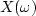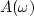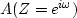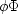Next: MATRICES AND VECTORS Up: Notation Previous: SCALARS

# FILTERS, SIGNALS, AND THEIR TRANSFORMS

The example x(t), xt, Xk, X(Z),can be understood as follows. A lower-case letter with a function argument (t) denotes a continuous time function (rare). Lower case with a subscript denotes a signal or filter as a function of discrete time (common). Upper case with subscript denotes a discrete Fourier transform. Z-transforms are denoted by the function argument (Z). Where a function argumentis occasionally seen, such as in,it is generally a shorthand for.For a definition of the complex conjugate of filters, see page.

aA 		feedback filter (autoregression)
bB 		convolution filter
cC 		causal filter; reflected wave; cross-spectrum
dD 		downgoing wave
E 		escaping wave
fF 		component of layer matrix; force; generic function
gG 		component of layer matrix; analytic signal; causal garbage filter
I 		causal integration operator
JKLMO 		unused
N 		noise
pP 		phase shift; pressure; all-pass filter; generic input space
qQ 		quadrature filter; generic output space
rR 		impedance; reflection seismogram
sS 		S is spectrum; st is autocorrelation
T 		transmitted wave
uU 		upcoming wave; logarithm of S
vV 		velocity
W 		weighting function; vertical component of flow
xX 		generic input signal
yY 		generic output signalphaseNext: MATRICES AND VECTORS Up: Notation Previous: SCALARS
Stanford Exploration Project
10/21/1998